Home > CC2MN > Chapter cc29 > Lesson cc29.1.1 > Problem9-14

9-14.
1. Janet sells erasers at a price of 8 erasers for 60 cents. She started this table to show the price for different numbers of erasers. 9-14 HW eTool (Desmos). Homework Help ✎

 Erasers 8 4 1 12 10 16 100 \$ 0.6
1. Complete her table.

2. Does this situation represent a proportional relationship? Why or why not?

3. Use the information in the table to find the cost of 144 erasers. Write and solve a proportion, if appropriate.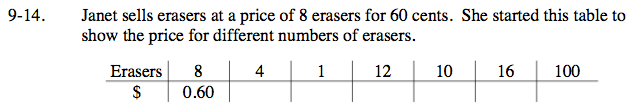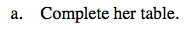Set up a proportion to help you fill in the table.

$\frac{8}{0.60}=\frac{4}{\textit{x}}$

Solve for x.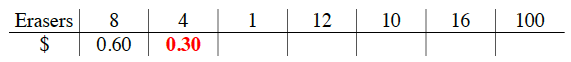Use the same method to fill in the rest of the table.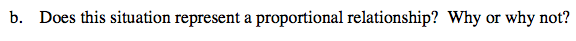Look at the values you found for the table.
Are the different values related? If they are, how are they related?
Does this tell you if they are proportional?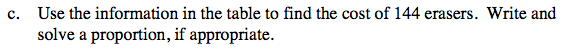Use the same method as part a to find the cost of 144 erasers.

Use the eTool below to solve the problem.
Click the link at right for the full version of the eTool: CC2 9-14 HW eTool